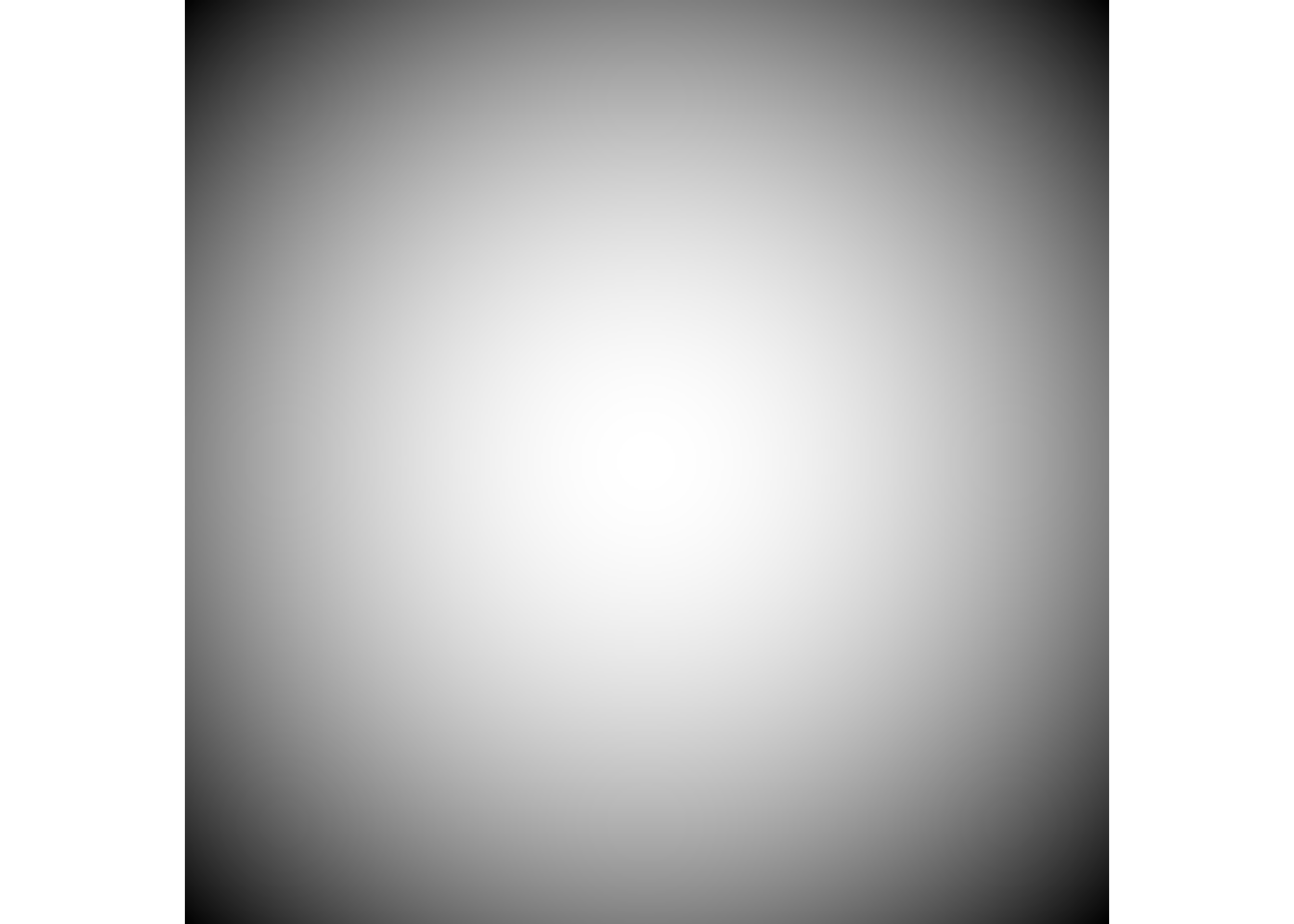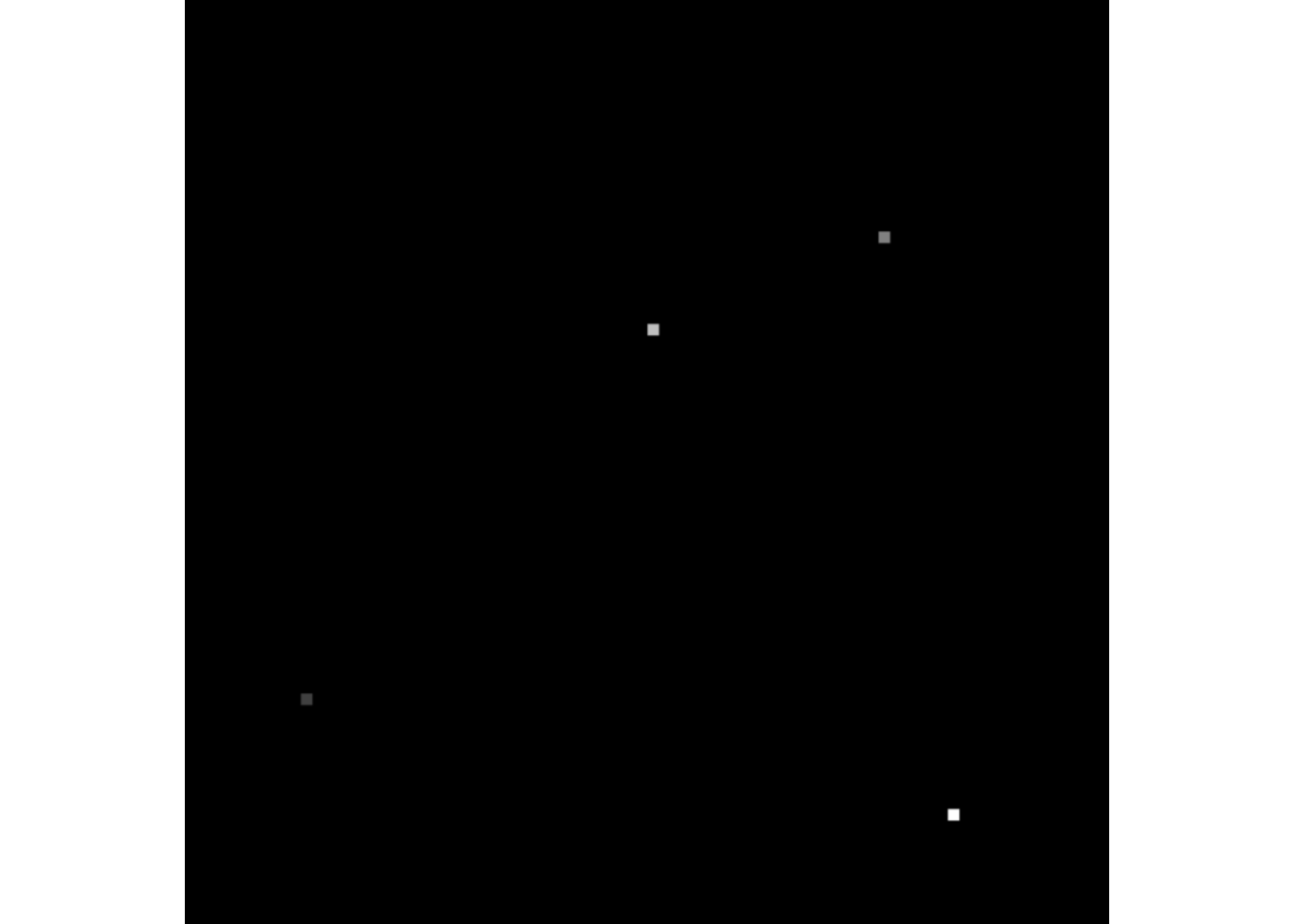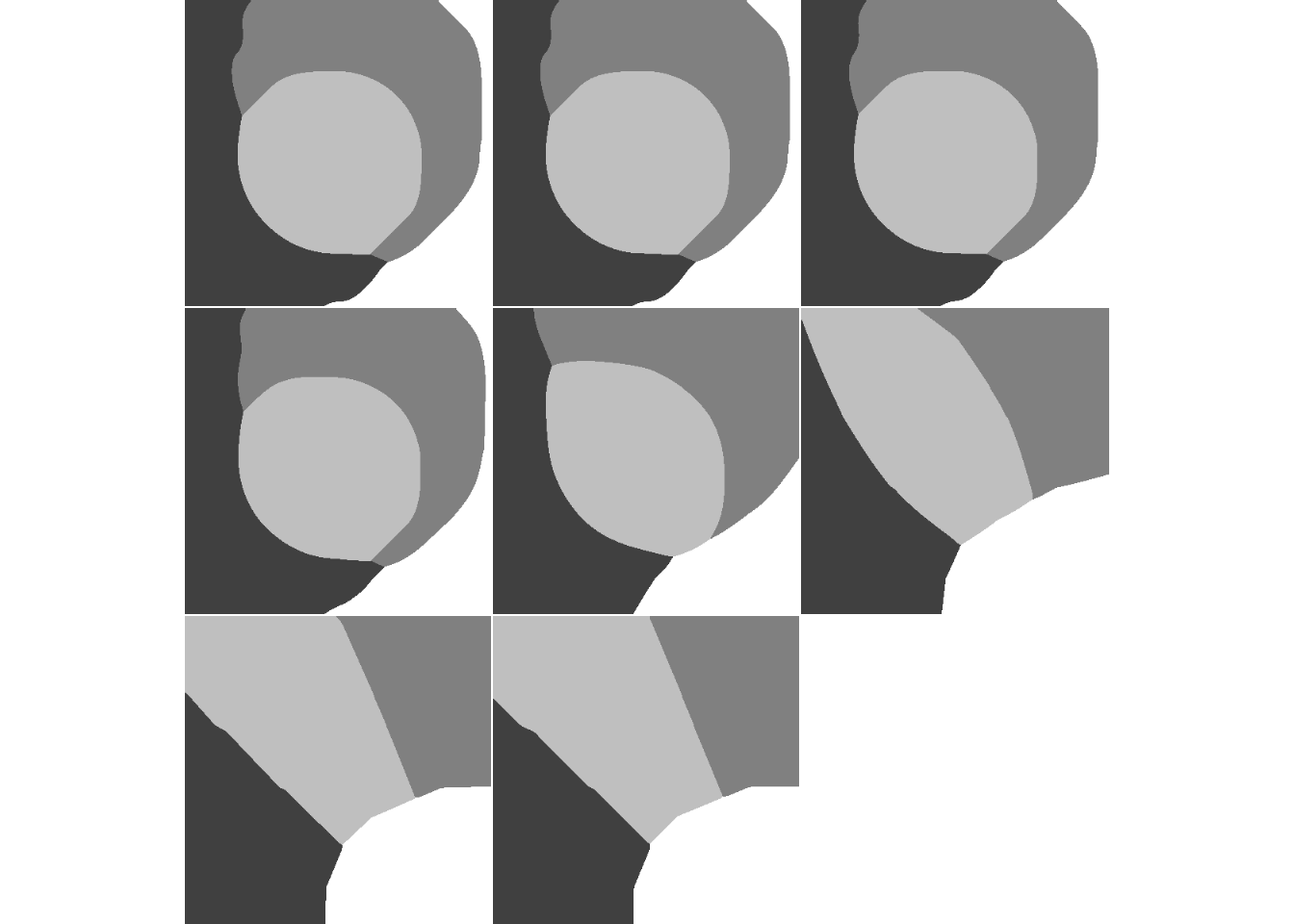RNotes | EBImage

library(EBImage)

# Propagate

Voronoi-based segmentation on image manifolds

## Paraboloid mountain in a plane

library(EBImage)

n <- 400
x <- (n/4)^2 - matrix(
(rep(1:n, times=n) - n/2)^2 + (rep(1:n, each=n) - n/2)^2,
nrow=n, ncol=n)
x <- normalize(x)

## 4 seeds

seeds <- array(0, dim=c(n,n))
seeds[51:55, 301:305] <- 1
seeds[301:305, 101:105] <- 2
seeds[201:205, 141:145] <- 3
seeds[331:335, 351:355] <- 4

lambda <- 10^seq(-8, -1, by=1)
segmented <- Image(dim=c(dim(x), length(lambda)))

for(i in seq_along(lambda)) {
prop <- propagate(x, seeds, lambda=lambda[i])
prop <- prop/max(prop)
segmented[,,i] <- prop
}

display(x, title='Image')display(seeds/max(seeds), title='Seeds')display(segmented, title="Voronoi regions", all=TRUE)The example produces a sequence of segmentation scenarios with varying lambda parameter controlling the weight of the Euclidean distance term in the Voronoi tessellation. The first image in the sequence corresponds to a situation when the segmentation is predominantly driven by the image content (lambda close to 0) while the last one shows a mostly Euclidean space division. For details see the references from ?propagate.

packageVersion("EBImage")
##  '4.8.2'

efg
2015-01-22 1241